# The evolution of the directors

Here, we explicitly show how, with knowledge of the strains for a directed curve and a particular parameterization of a rotation tensor, one may determine the orientation of the curve’s associated directors at each material point by solving a system of ordinary differential equations.

## Equations of motion

Referring to Figure 1, consider an inextensible material curve of length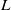with convected coordinate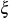. We define a right-handed orthonormal triad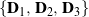at each material point in the curve’s reference configuration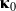. Similarly, we have at eachin the curve’s current configuration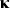an associated triad, where the vectorsand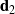are known as directors. Note that the absolute position of a material point on the curve in the reference and current configurations is denoted by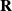and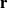, respectively, which are expressed in terms of the space-fixed basis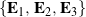.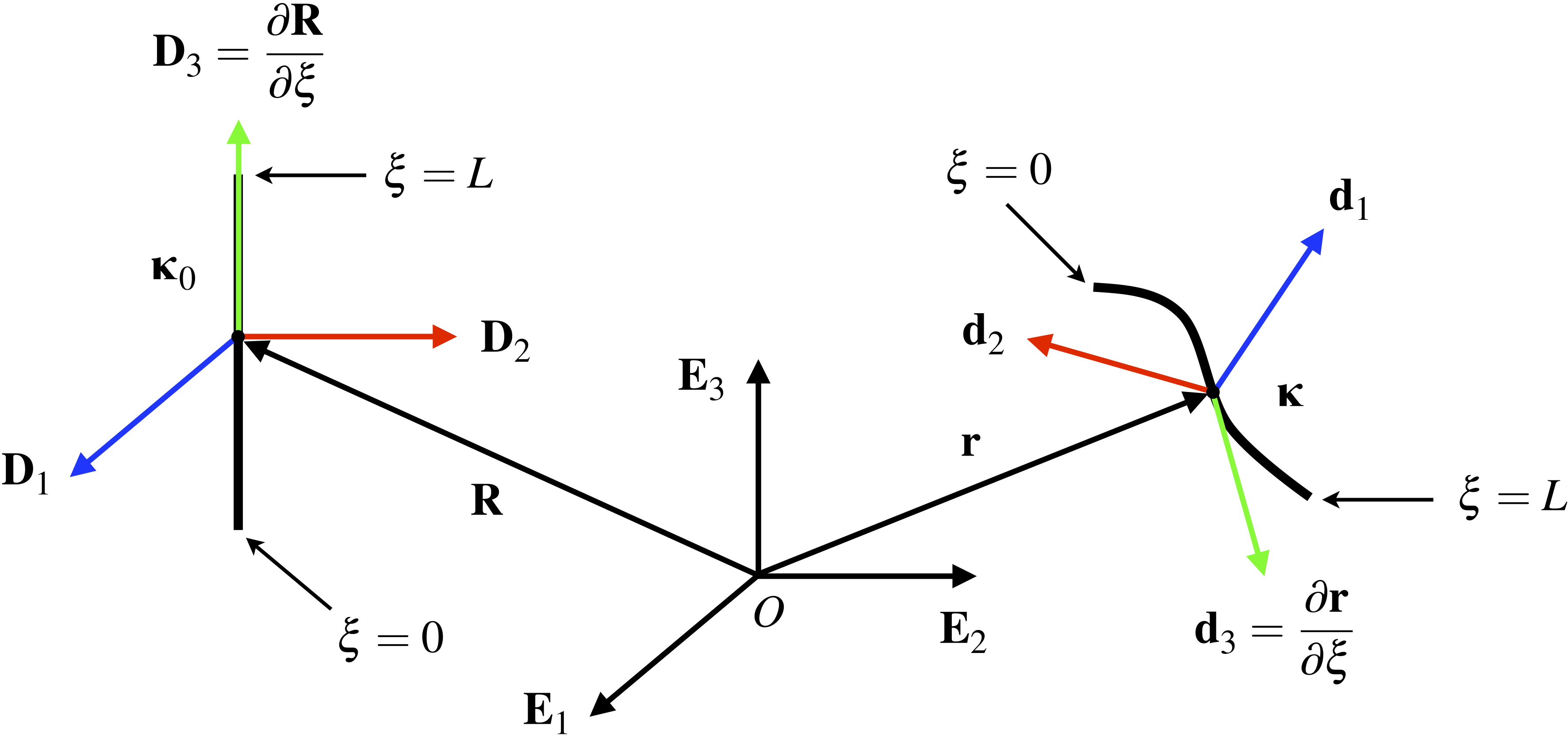Figure 1. Schematic of a directed curve in the reference and current configurations, and , respectively.

Recall that according to Kirchhoff’s rod theory, the triadsandat eachfor the material curve are related such that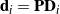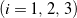, where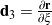,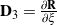, and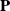is a rotation tensor. As illustrated in Figure 1, we assume the material curve is undeformed (i.e., it is straight with no pre-twist) in the reference configurationsuch that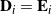.

Following Love , we use a 3-2-3 set of Euler angles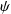,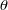, and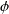to parameterize the rotation tensor, and therefore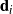and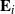are related via the matrix-vector expression

(1)Kirchhoff’s constraints imply that there exists an axial vector

(2)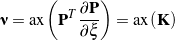associated with the skew-symmetric tensor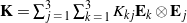, with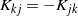for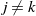. Consequently, (2) yields

(3)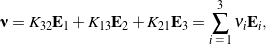where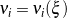denote strain measures for the material curve;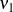and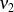correspond to bending of the curve, while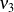is associated with torsion. By extracting thecomponents of expression (3), we obtain a system of ordinary differential equations that governs the orientation of the triadat every material point on the directed curve. These differential equations may be expressed in the first-order form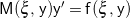for numerical integration in MATLAB, where the prime denotes differentiation with respect to the convected coordinate. If we define the state vector, then

(4)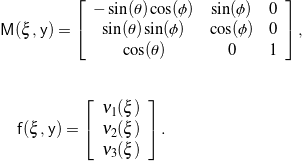Thus, by providing the strainsand the initial orientation of the directed curve, we may determine the evolution of the Euler angles, and hence the orientation of, along the curve. Lastly, because, the shape of the material curve,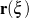, is obtained as follows:

(5)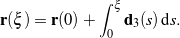## Simulation and animation

For the simulation results that follow, the material curve is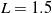m long and begins at the origin: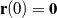. The initial orientation was chosen such that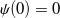,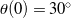, and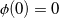in order to avoid a singularity in the 3-2-3 set of Euler angles.

First, as a check of our numerical simulation, suppose we leave the material curve undeformed, i.e., the strains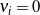. In this case, we expect the triadto remain in the same orientation at each material point along the curve. This behavior is verified when we animate the corresponding results from numerical integration; a sample animation is provided in Figure 2. In this and subsequent animations, the directorsandare drawn in blue and red, respectively, while the vector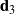is drawn in green, as shown in Figure 1.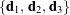Figure 2. Animation of the triad along an undeformed directed curve: .

Next, we may cause the material curve to be in pure bending by prescribing either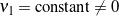,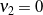, and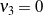, or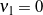,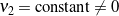, and. The animation in Figure 3 demonstrates the variation inalong the curve for the case when,, and.Figure 3. Animation of the triad along a directed curve subject to pure bending: , , and .

Pure torsion of the material curve is possible if we set,, and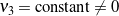. For example, when we prescribe,, and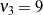, it is clear from the corresponding animation in Figure 4 that the spinning behavior ofresults from the curve having been twisted.Figure 4. Animation of the triad along a directed curve subject to pure torsion: , , and .

Lastly, we may deform the material curve in more complicated ways by combining bending and torsion, and by specifying strains that vary along the curve. Consider, for example, the material curve depicted in Figure 5 that is subject to both constant bending and torsion: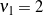,, and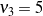. Consequently, the variation inalong the curve is more elaborate than the cases of pure bending and pure torsion.Figure 5. Animation of the triad along a directed curve subject to constant bending and torsion: , , and .

Alternatively, suppose the strains vary along the material curve according to, say,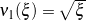,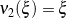, and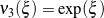. As shown in Figure 6, the orientation ofat each material point varies in a more complicated manner than for the simple bending or torsion cases.Figure 6. Animation of the triad along a directed curve with variable strains: , , and .